# Probability & Permutation and Combination Quiz for IBPS, SBI, RBI: Quiz – 8

## Probability & Permutation and Combination Quiz for IBPS, SBI, RBI: Quiz – 8

Probability and Permutation & Combination is a significant topic for all Banking exams. Probability and Permutation & Combination is a topic that is frequently asked in every competitive exam. So here we are providing you the Probability and Permutation & Combination Quiz with a full explanation for each question. This Probability and Permutation & Combination Quiz is based on the latest or updated exam pattern. Candidates should solve this Probability and Permutation & Combination Quiz in order to gain a better understanding of the topic and acquire methods that help them answer more tricky questions.

1. A bag contains 31 cards with numbered 1, 2, 3, ……., 31. Two cards are picked at random (one after another and without replacement). Find the probability that the sum of numbers of both cards is odd.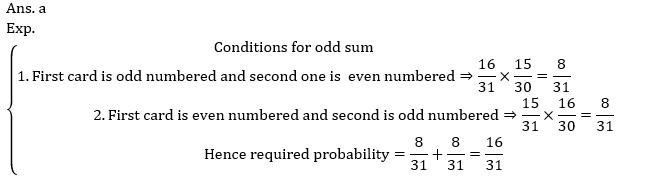2. A bag contains 24 eggs out of which 8 are rotten. The remaining eggs are not rotten eggs. Two eggs are selected at random, what is the probability that at least one of the eggs is rotten ?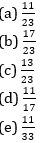3. A bag contains 3 red balls, 5 yellow balls and 7 pink balls. If one ball is drawn at random from the bag, what is the probability that it is either pink or red?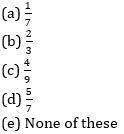4. A three-digit number is chosen at random. What is the probability that all the digits are distinct, the digits at odd places are odd and the digits at even places are even?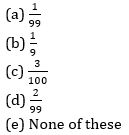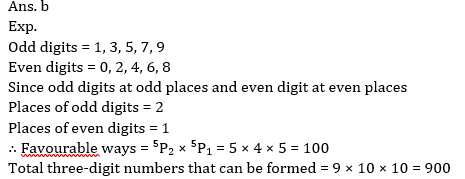5. A box contains 5 blue, 3 green and 4 red balls. 2 balls are drawn from the box at random. What is the probability that both the balls are of the same colour ?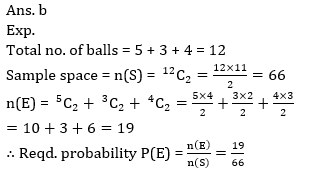6. Find the total arrangement of the words ‘ALLAHABAD’ such that vowels do not come together?
(a) 6800
(b) 7200
(c) 6250
(d) 7650
(e) 82007. A number is selected at random from the first 35 natural numbers. What is the probability that it is either a multiple of 5 or a multiple of 7?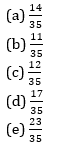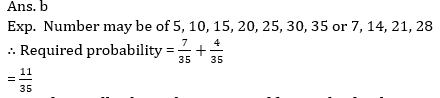8. A fruit seller have three types of fruit in his bucket namely Mango, Orange and Papaya. The probability of selling one mango is 2/7 and one orange is 1/6. If seller have total 46 papaya in his bucket then find total number of fruits in seller bucket ?
(a) 78
(b) 84
(c) 96
(d) 80
(e) 72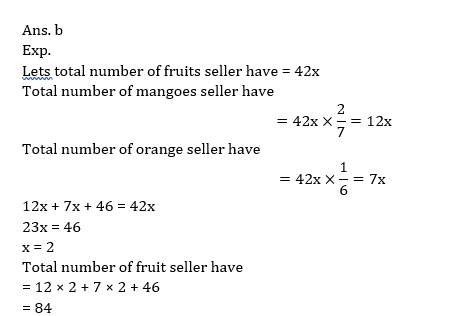9. In a bag 6 Math books, 4 English books and 8 Science books. If three books are picked up at random, find the probability that one is Math books and two are Science books ?10. A bag contains some red, blue and green color marbles. The probability of selecting one red marble out of total marbles is 3/10 and the probability of selecting one green marble out of total marbles is 2/5. The number of blue colour marbles in the bag is 6. Find probability of selecting three marbles such that at most two marbles are of same color?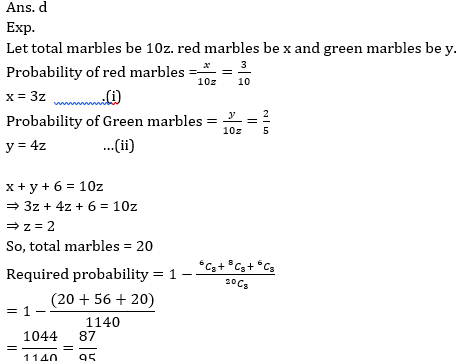#### Attempt Quantitative Aptitude Topic Wise Online Test Series

Recommended PDF’s for:

#### Most important PDF’s for Bank, SSC, Railway and Other Government Exam : Download PDF Now

AATMA-NIRBHAR Series- Static GK/Awareness Practice Ebook PDF Get PDF here
The Banking Awareness 500 MCQs E-book| Bilingual (Hindi + English) Get PDF here
AATMA-NIRBHAR Series- Banking Awareness Practice Ebook PDF Get PDF here
Computer Awareness Capsule 2.O Get PDF here
AATMA-NIRBHAR Series Quantitative Aptitude Topic-Wise PDF Get PDF here
AATMA-NIRBHAR Series Reasoning Topic-Wise PDF Get PDF Here
Memory Based Puzzle E-book | 2016-19 Exams Covered Get PDF here
Caselet Data Interpretation 200 Questions Get PDF here
Puzzle & Seating Arrangement E-Book for BANK PO MAINS (Vol-1) Get PDF here
ARITHMETIC DATA INTERPRETATION 2.O E-book Get PDF here
3